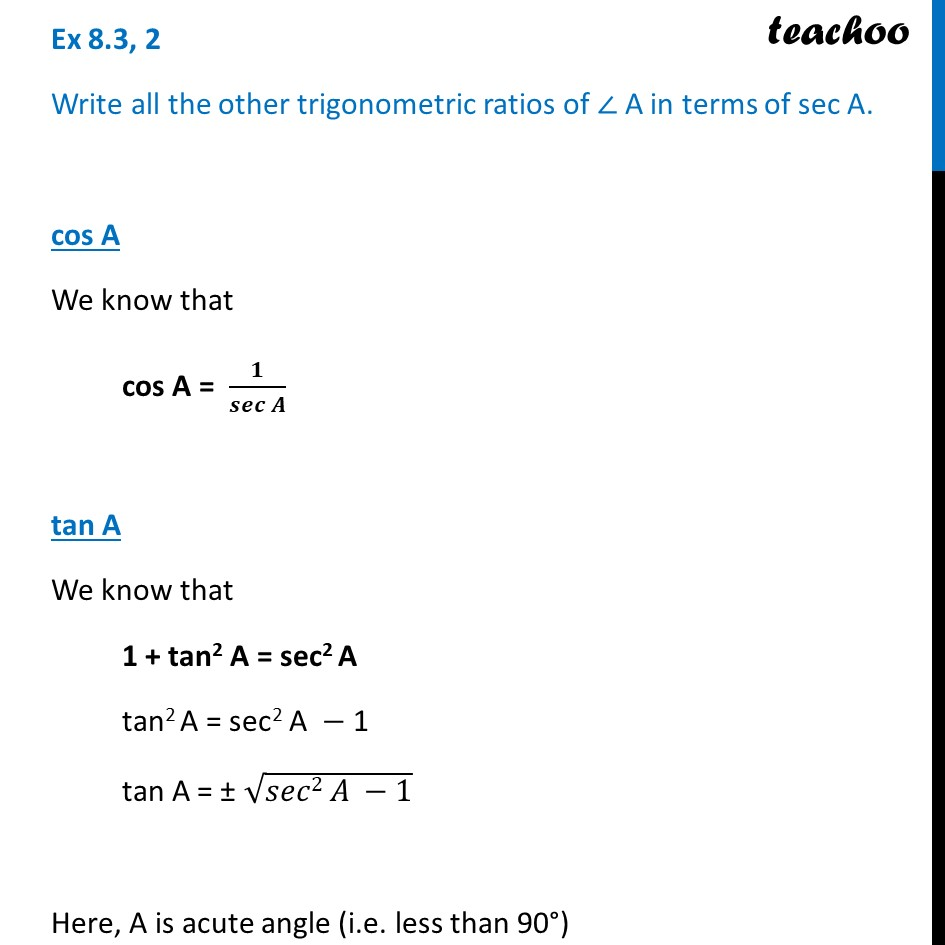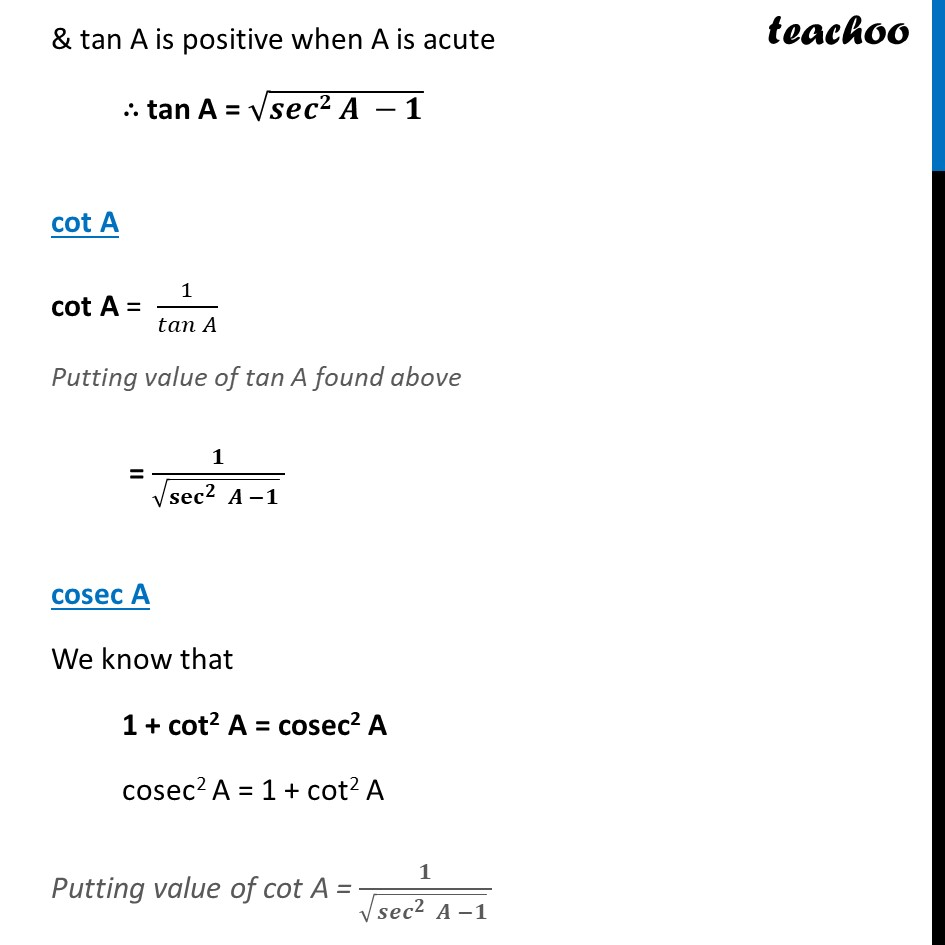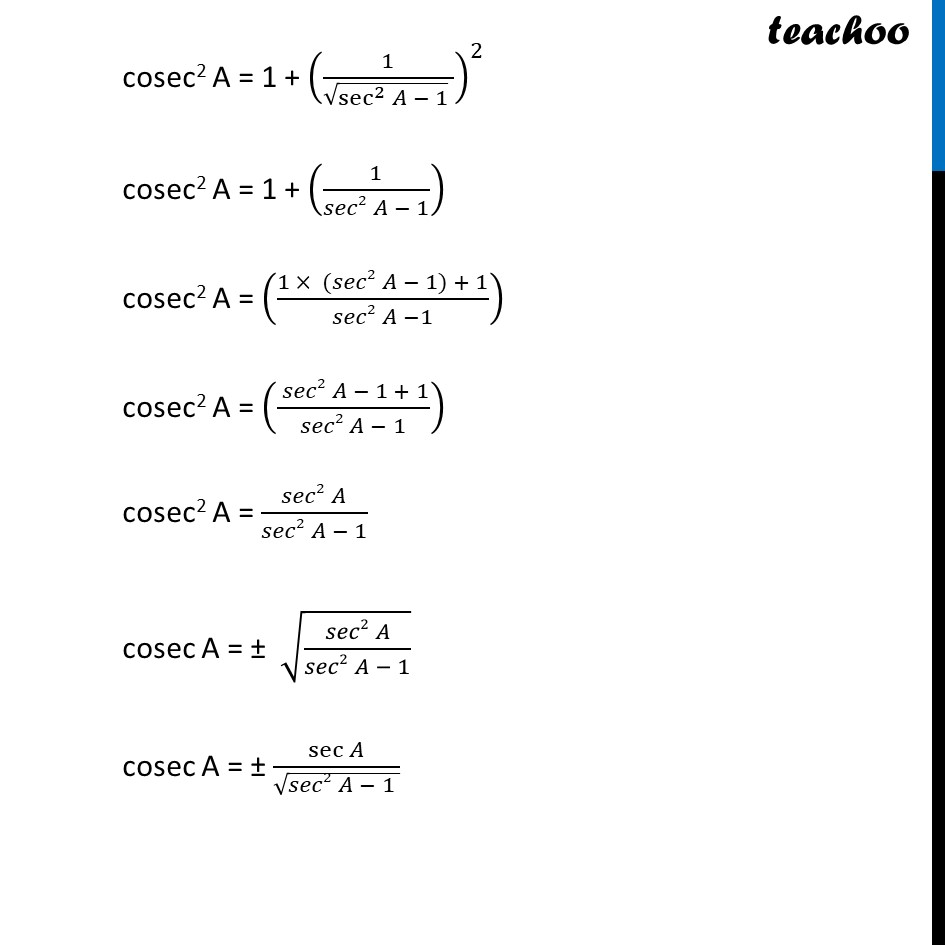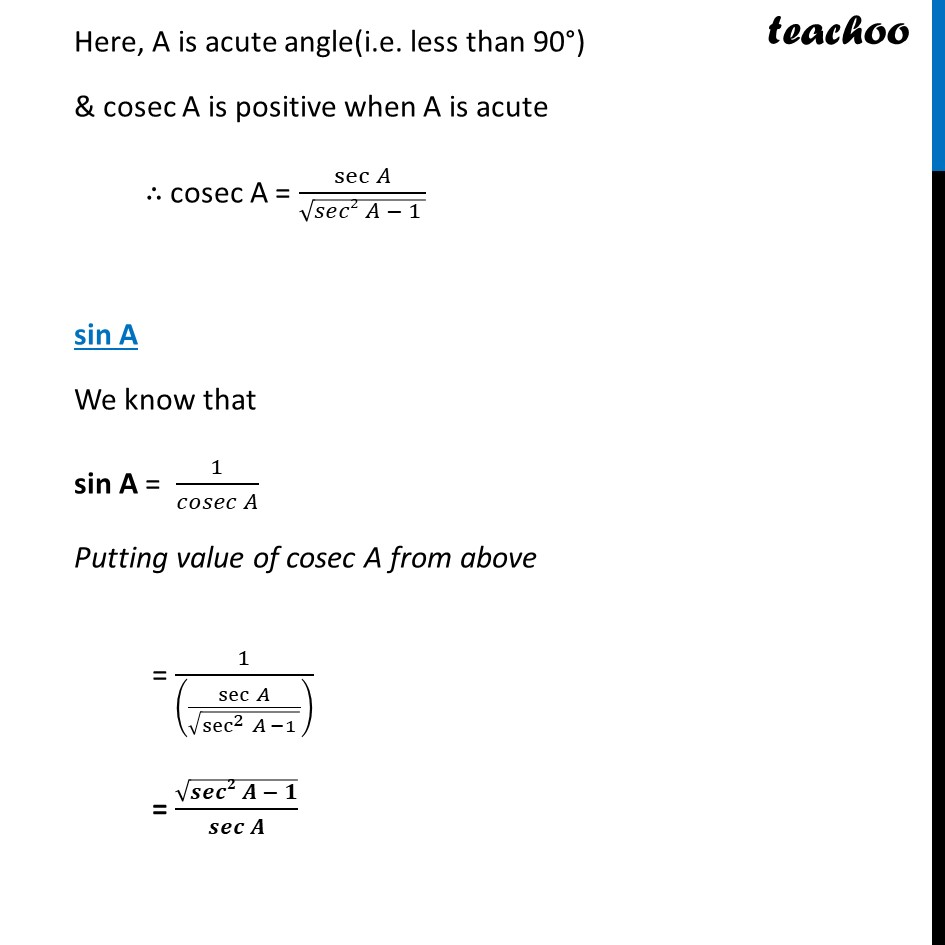Ex 8.3

Chapter 8 Class 10 Introduction to Trignometry
Serial order wiseLearn in your speed, with individual attention - Teachoo Maths 1-on-1 Class

### Transcript

Ex 8.3, 2 Write all the other trigonometric ratios of ∠ A in terms of sec A. cos A We know that cos A = 𝟏/𝒔𝒆𝒄⁡𝑨 tan A We know that 1 + tan2 A = sec2 A tan2 A = sec2 A − 1 tan A = ± √(𝑠𝑒𝑐2 𝐴 −1) Here, A is acute angle (i.e. less than 90°) & tan A is positive when A is acute ∴ tan A = √(𝒔𝒆𝒄𝟐 𝑨 −𝟏) & tan A is positive when A is acute ∴ tan A = √(𝒔𝒆𝒄𝟐 𝑨 −𝟏) cot A cot A = 1/(𝑡𝑎𝑛 𝐴) Putting value of tan A found above = 𝟏/(√(〖𝐬𝐞𝐜〗^𝟐⁡〖 𝑨 −𝟏〗 ) ) cosec A We know that 1 + cot2 A = cosec2 A cosec2 A = 1 + cot2 A Putting value of cot A = 𝟏/(√(〖𝒔𝒆𝒄〗^𝟐⁡〖 𝑨 −𝟏〗 ) ) cosec2 A = 1 + (1/(√(sec^2⁡〖𝐴 − 1〗 ) ))^2 cosec2 A = 1 + (1/(𝑠𝑒𝑐2 𝐴 − 1)) cosec2 A = ((1 × (𝑠𝑒𝑐2 𝐴 − 1) + 1)/(𝑠𝑒𝑐2 𝐴 −1)) cosec2 A = (( 𝑠𝑒𝑐2 𝐴 − 1 + 1)/(𝑠𝑒𝑐2 𝐴 − 1)) cosec2 A = ( 𝑠𝑒𝑐2 𝐴 )/(𝑠𝑒𝑐2 𝐴 − 1) cosec A = ± √(( 𝑠𝑒𝑐2 𝐴 )/(𝑠𝑒𝑐2 𝐴 − 1)) cosec A = ± sec⁡𝐴/√(𝑠𝑒𝑐2 𝐴 − 1 ) Here, A is acute angle(i.e. less than 90°) & cosec A is positive when A is acute ∴ cosec A = sec⁡𝐴/√(𝑠𝑒𝑐2 𝐴 − 1 ) sin A We know that sin A = 1/(𝑐𝑜𝑠𝑒𝑐 𝐴) Putting value of cosec A from above = 1/((sec⁡𝐴/√(sec^2⁡〖𝐴 −1〗 )) ) = √(𝒔𝒆𝒄𝟐 𝑨 − 𝟏)/𝒔𝒆𝒄⁡𝑨